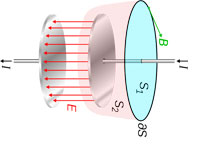#Difference Between Similar Terms and Objects

# Difference Between Electric field and Electric PotentialElectric field vs Electric potential

An electric field is present around a charge – either negative or positive. Any charged object can also acquire that field of electric force. A charge or a charged object has a force of whether to attract or repel a surrounding charge or object. Surrounding charges have also electric fields of their own with varying magnitude and this must also be taken into account.

The electric field is a force that acts between two charges and it is somewhat analogous to the gravitational field force that acts between two masses. The only difference is that gravitational force will be dependent on the values of masses while electric force will depend on the amount of charges on objects.

The formula for an electric field is as follows:

E = K * Q/d^2

Where:

K = constant
Q = charge in unit Coulomb (C)
d = distance between a charged object in unit meter (m)

Electric field is measured in Newtons per Coulomb which means that the field intensity of an Electric Field (E) is described as the amount of force (F) present for every coulomb of charge. The vector quantity can also be expressed in the unit of Volts per Meter.

Thus,

E = F/Q

This is a vector quantity that will either go in the direction of attraction or repulsion. It should be noted that a test charged should applied to get the Electric field intensity (E) of an object as there is no way to know how intense the field is, if it acts alone. In shorter terms, “It takes one to know one.”

The total amount of work to be done to move a charge from one place to another without causing any acceleration is what we call as the Electric Potential. The electric potential is the potential energy of a unit of charge that is associated with a static — time-invariant — electric field.

Mathematically, it is described as,

Ve = W/Q

The electric potential (Ve) is expressed in volts or Joules per Coulomb. Joules is the a unit of Work and as the formula shows, electric potential (Ve) is the amount of Work (W) per unit charge (Q). This quantity is scalar quantity which is often symbolized by a non-bolded V to represent its scalar property.

Summary:
1. Electric field is described as the amount of force per charge while the Electric potential is described as the amount of energy or work per charge.
2. Electric field is measured in Newtons per Coulomb or Volts per meter while Electric Potential is measured in unit Volts or Joules per Coulomb
3. Electric field is a vector quantity while Electric potential is a scalar quantity.

Latest posts by Ian (see all)

### Search DifferenceBetween.net :

Custom Search

Help us improve. Rate this post!(10 votes, average: 2.50 out of 5)Loading...Email This Post : If you like this article or our site. Please spread the word. Share it with your friends/family.

Please note: comment moderation is enabled and may delay your comment. There is no need to resubmit your comment.

Articles on DifferenceBetween.net are general information, and are not intended to substitute for professional advice. The information is "AS IS", "WITH ALL FAULTS". User assumes all risk of use, damage, or injury. You agree that we have no liability for any damages.

See more about : , , ,